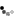# Please solve this problem for me?

India
February 16, 2009 12:50pm CST
I read this somewhere I don't remember, was some magazine, not too sure. I remember the question distinctly but kinda forgot the answer. I mean I tried to memorize the digits but lost it. The Question was: There is this weight measuring 40kgs, which has been broken into four parts. Now, one must be able to measure any weight between 1 to 40kgs using a combination of these four parts. Also the weight of all the four parts put together must equal 40 kgs. What are the weights of the four parts? This ain't a trick question and the answer definitely exists. Can someone help me with this please?
2 responses
@exinco (214)
• Malaysia
16 Feb 09
what is this question? quiz? lost weight tips?
• India
16 Feb 09
Did you not understand the question. I have labeled it 'Quiz' now. And it's definitely not weight loss.
• United States
16 Feb 09
Correct me if I'm wrong, but the numbers would be 1, 3, 9,and 27. 1 = 1 (obviously), 3-1=2, 3=3, 3+1=4, 9-3-1=5, 9-3=6, 9+1-3=7, etc. I haven't made it up through 40 quite yet, but I'll let you know when I do ;)!
1 person likes this• Philippines
17 Feb 09
There is no definite answer to your question bro. There are a lot of possible combination of 4 numbers that sum up to 40. Either be 10, 10, 10, & 10; or 1, 1, 1, and 37; or 5, 5, 5, and 25. Countless options. I believe there is something missing in the question. Maybe proportions from the first number to the second number and the 2nd number to the 3rd and the 3rd to the 4th.. Something like that. Solving such kind of mathematical problems would be easy provided the data provided in the problem is sufficient.
• India
18 Feb 09
The data provided in the problem is sufficient, and I assure you that nothing is missing from the problem. Happy Quizzing!